Refractive Index of Glass slab using Travelling Microscope

# Our Objective

To determine refractive index of a glass slab using a travelling microscope.

# The Theory

When a ray of light travelling in a straight line in a transparent homogenous medium encounters another transparent medium, it bends at the boundary of the two media and then travel again on a straight line in the other medium. This phenomenon of bending of a ray of light under oblique incidence at a boundary or interface of two optical media is called refraction of light.

Snell’s law of refraction:

According to this law, the ratio of the sine of the angle of incidence to the sine of angle of refraction is constant.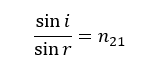where n21 is a constant, called the refractive index of the second medium with respect to the first medium.

Refractive index is a characteristic of the pair of media that depends on the wavelength of light, but is independent of the angle of incidence. It is a dimensionless quantity and is always calculated with respect to another medium. The refractive index can be linked to the relative speed of propagation of light in different media. The refractive index of medium 2 with respect to medium 1 (n21) is given by the ratio of the speed of light in medium 1 and the speed of light in medium 2.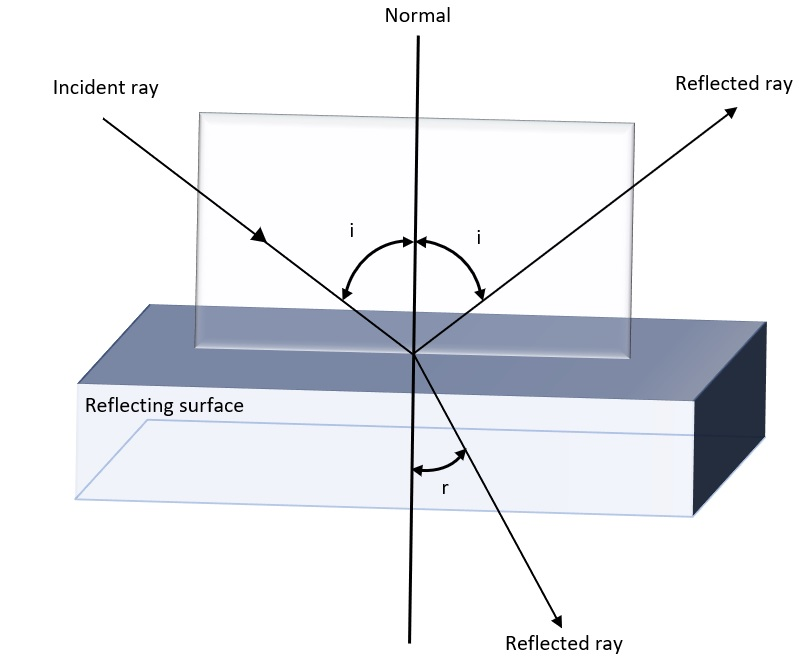If medium 2 is optically denser than medium 1, n21 > 1, r < i , i.e., the refracted ray bends towards the normal. On the other hand, when incident ray in a denser medium refracts into a rarer medium n21 <1, r > i, and the refracted ray bends away from the normal.

Hence, when an object placed in optically denser medium is viewed from a rarer medium, it appears to be at a depth less than the real depth due to refraction. For viewing near the normal direction, the ratio of real depth to the apparent depth of a refracting medium gives the refractive index of the medium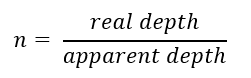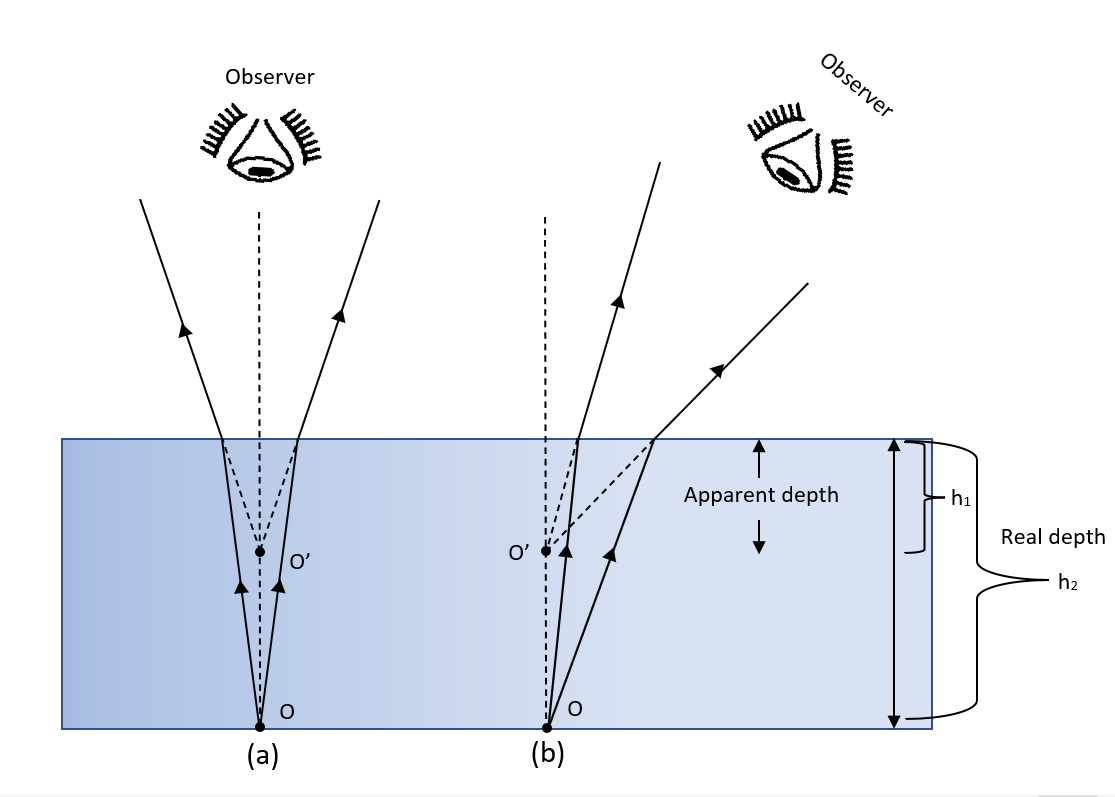When a point O at the bottom of the glass slab is observed through a glass slab appears to come from O’. O’ is the virtual image of the real object O formed on the normal. The distance between this apparent bottom and the top surface of the slab gives the apparent thickness of the slab (h1). The apparent depth or thickness (h1) of the glass slab is less than the real depth (h2).

The refractive index of glass with respect to the medium that is air is given as: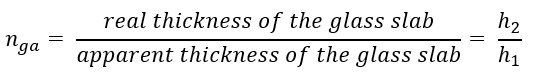A travelling microscope can be used to find the real and apparent thickness of the glass slab and hence calculate its refractive index.

# Learning Outcomes

• Students gain a better understanding of refraction through glass slab.
• Students learn a method to find refractive index of a glass slab.
• Students learn to relate refractive index of a material to its apparent depth.
• Students learn to handle a travelling microscope.